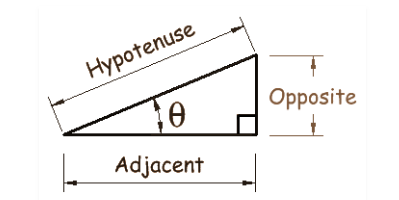# Sin Cos Formulas

Sin Cos formulas are based on the sides of the right-angled triangle. Sin and Cos are basic trigonometric functions along with tan function, in trigonometry. The sine of an angle is equal to the ratio of the opposite side to the hypotenuse whereas the cosine of an angle is equal to the ratio of the adjacent side to the hypotenuse.

• Sin θ = Opposite side/Hypotenuse
• Cos θ = Adjacent side/ Hypotenuse## Basic Trigonometric Identities for Sin and Cos

These formulas help in giving a name to each side of the right triangle and these are also used in trigonometric formulas for class 11. Let’s learn the basic sin and cos formulas.

• cos2(A) + sin2(A) = 1

## Sine and Cosine Formulas

To get help in solving trigonometric functions, you need to know the trigonometry formulas.

### Half-angle formulas

Sin
$$\begin{array}{l}\frac{A}{2}\end{array}$$
=
$$\begin{array}{l}\pm \sqrt{\frac{1- Cos A}{2}}\end{array}$$
• If A/2 is in the first or second quadrants, the formula uses the positive sign.
• If A/2 is in the third or fourth quadrants, the formula uses the negative sign
Cos
$$\begin{array}{l}\frac{A}{2}\end{array}$$
=
$$\begin{array}{l}\pm \sqrt{\frac{1+ Cos A}{2}}\end{array}$$
• If A/2 is in the first or fourth quadrants, the formula uses the positive sign.
• If A/2 is in the second or third quadrants, the formula uses the negative sign

### Double and Triple angle formulas

• Sin 2A = 2Sin A Cos A
• Cos 2A = Cos2A – Sin2A = 2 Cos2 A- 1 = 1- Sin2A
• Sin 3A = 3Sin A – 4 Sin 3A
• Cos 3A = 4 Cos3A – 3CosA
• Sin2A =
$$\begin{array}{l}\frac{1 – Cos(2A)}{2}\end{array}$$
• Cos2A =
$$\begin{array}{l}\frac{1 + Cos(2A)}{2}\end{array}$$

### Sum and Difference of Angles

• sin(A + B) = sin(A).cos(B) + cos(A)sin(B)
• sin(A−B)=sin(A)⋅cos(B)−cos(A)⋅sin(B)
• cos(A+B)=cos(A)⋅cos(B)−sin(A)⋅sin(B)
• cos(A−B)=cos(A)⋅cos(B)+sin(A)⋅sin(B)
• sin(A+B+C)=sinA⋅cosB⋅cosC+cosA⋅sinB⋅cosC+cosA⋅cosB⋅sinC−sinA⋅sinB⋅sinC
• cos (A + B +C) = cos A cos B cos C- cos A sin B sin C – sin A cos B sin C – sin A sin B cos C
• Sin A + Sin B = 2Sin
$$\begin{array}{l}\frac{(A+B)}{2}\end{array}$$
Cos
$$\begin{array}{l}\frac{(A-B)}{2}\end{array}$$
• Sin A – Sin B = 2Sin
$$\begin{array}{l}\frac{(A-B)}{2}\end{array}$$
Cos
$$\begin{array}{l}\frac{(A+B)}{2}\end{array}$$
• Cos A + Cos B = 2Cos
$$\begin{array}{l}\frac{(A+B)}{2}\end{array}$$
Cos
$$\begin{array}{l}\frac{(A-B)}{2}\end{array}$$
• Cos A – Cos B = -2Sin
$$\begin{array}{l}\frac{(A+B)}{2}\end{array}$$
Sin
$$\begin{array}{l}\frac{(A-B)}{2}\end{array}$$

### Multiple Angle Formulas

Sin (2θ) = 2 sin θ cos θ

Cos (2θ) = cos2θ – sin2θ =2 cos2θ -1 = 1- 2 sin2θ

### Product To Sum Formulas

2 cos θ cos φ = cos (θ-φ) + cos (θ+φ)

2 sinθ sin φ = cos (θ-φ) – cos (θ+φ)

2 sin θ cos φ = sin  (θ+φ) + sin (θ-φ)

2 cos θ sin φ = sin (θ+φ) – sin (θ-φ)

### Periodic Identities

 Sin ((π/2) – x) = cos x Cos ((π/2) – x) = sin x Sin ((π/2) + x) = cos x Cos ((π/2) + x) = -sin x Sin ((3π/2) – x) = -cos x cos ((3π/2) – x) = -sin x Sin ((3π/2) + x) = -cos x Cos ((3π/2) + x) =  sin x Sin (π – x) = sin x Cos (π – x) = – cos x Sin (π + x) = -sin x Cos (π + x) = -cos x Sin (2π – x) = -sin x Cos (2π – x) = cos x Sin (2π + x) = sin x Cos (2π + x) = cos x

### Sine Law

$$\begin{array}{l}\frac{\sin A}{a}=\frac{\sin B}{b}=\frac{\sin C}{c}\end{array}$$

### Cosine Law

a2 = b2+c2 – 2bc cos A

b2 = c2+ a2 – 2ca cos B

c2 = a2+b2 – 2ab cos C

### Product Identities

Sin x cos y = (½)[sin (x+y) + sin (x-y)]

Cos x  sin y = (½) [sin (x+y)- sin (x-y)]

Cos x cos y = (½)[cos(x-y) + cos (x+y)]

Sin x sin y = (½) [cos (x-y) – cos (x+y)]

### Example on Sin Cos Formula

Example:

Find the value of sin 20° sin 40° sin 60° sin 80°.

Solution:

Given: sin 20° sin 40° sin 60° sin 80°

This can be written as:

⇒ sin 60° sin 20° sin 40° sin 80°

Substitute sin 60° = √3/2

⇒ (√3/2) sin 20° (sin 60°-20°) sin (60°+20°)

⇒ (√3/2) sin 20° (sin2 60° – sin2 20°)

⇒ (√3/2)(¼) [3 sin 20° – 4 sin3 20°]

Simplifying 3 sin 20° – 4 sin3 20°, we get sin 60°

Now substitute the values,

⇒(√3/2)(¼)(√3/2)

⇒(¾)(¼)

⇒ 3/16

Hence, the value of sin 20° sin 40° sin 60° sin 80° is 3/16.